×
Get Full Access to Carleton University - PHYS 1008 - Class Notes - Week 2
Get Full Access to Carleton University - PHYS 1008 - Class Notes - Week 2

×

# electric field symbol Description

##### Description: These notes cover lecture 2 of the course work.
39 Pages 302 Views 1 Unlocks
Reviews

## (c) What is the charge on the sphere?## (b) What is the tension in the thread?## (a) What is the angle that the thread makes with the vertical?PHYS 1008ELEMENTARY UNIVERSITY PHYSICS II Instructor: Dr. Wafia Bensalem Textbook & Reference: Physics, Giambattista, Richardson, Richardson,  McGraw Hill, 2016.CHAPTER 1Electric Forces and Fields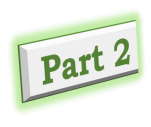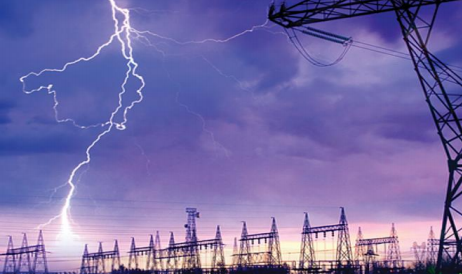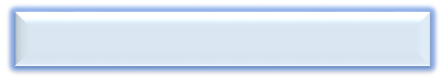4. THE ELECTRIC FIELD If a point charge q is in the vicinity of other charges, it  experiences an electric force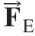.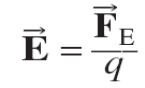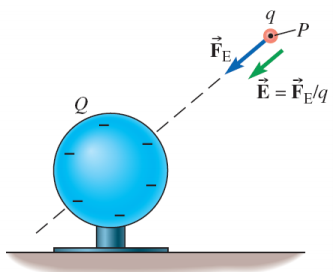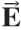The electric field (symbol ) at any point is defined to be  the electric force per unit  charge at that point. NB:is a vector quantityOnce we know the electric field at some point  it is easy to calculate the electric force on  any point charge q placed there: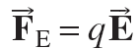Example 16.5 A small sphere of mass 5.10 g is  hanging vertically from an insulating  thread that is 12.0 cm long. By  charging some nearby flat metal  plates, the sphere is subjected to a  horizontal electric field of magnitude  7.20 × 105 N/C. As a result, the sphere is displaced 6.00 cm horizontally in  the direction of the electric field.  (a) What is the angle that the thread makes with the  vertical?  (b) What is the tension in the thread?  (c) What is the charge on the sphere?Slide 5 Strategy  • The electric force on the sphere is given by .  The figure shows that the sphere is pushed to the right  by the field; therefore, is to the right.  • Since and have the same direction, the charge on  the sphere is positive.  • After drawing an FBD showing all the forces acting on the  sphere, we set the net force on the sphere equal to zero  since it hangs in equilibrium.Solution (a)Solution (b)Solution (c)Electric Field due to a Point Charge What is the electric field [due to a single point charge Q] at a  position P, when P is at a distance r from Q Let q be a positive test charge placed at P.  Coulomb’s law says that the force acting on the test charge  has a magnitude: The electric field strength at P is then:Electric field strength at P Source charge Coulomb’s constantDistance between P and the source charge ❖ Principle of Superposition The electric field at any point is the vector sum of the field  vectors at that point caused by each charge separately. QUIZ 4 True or false? A charge of +3µC is at a point P where the electric field is directed to the  right and has a magnitude of 4 x 106 N/C.  If the charge is replaced with a -3µC charge, electric field at P will be  directed to the left.ANSWER False. Nothing happens to the electric field. It is created not by the  charge put on the point P, but by the source charge.Electric Field Lines Electric field lines are a set of continuous lines that  represent the electric field vector in space. • The direction of the electric field vector at any point is  tangent to the field line  passing through that point  and in the direction indicated  by arrows on the field line. • The electric field is strong where  field lines are close together and  weak where they are far apart.Rules for Sketching Field Lines • Electric field lines can start only on positive charges and  can end only on negative charges. • The number of lines starting on a positive charge (or  ending on a negative charge) is proportional to the  magnitude of the charge.  (The total number of  lines you draw is  arbitrary; the more lines  you draw, the better the representation of  the field.)• Field lines never cross. The electric field at any point has  a unique direction; if field lines crossed, the field would  have two directions at the same point. How to observe them?  Small pieces of thread on an oil surface align with the electric field.Field Lines for a Point Charge • The electric field due to a point charge is radial. It goes  away from a positive charge or toward a negative charge. • The lines are close together near the point charge, where  the field is strong, and are more spread out farther from  the point charge, showing that the field strength  diminishes with distance.QUIZ 5If E1 is the magnitude of the electric field at the  point P due to Q1 and E2 is the magnitude of the  electric field at the point P due to Q2; the net  electric field at the point P is: a) E1+ E2 b)22 2 E1+ E   c)E i E j 1+ 2   d)E j E i 1− 2 Q2(-) P X Q1(+) ANSWER D. The net electric field is the vector sum of the two electric fields due  to Q1 And Q2. Adding vectors does NOT mean adding magnitudes!  The electric field is a vector (NOT a scalar) quantity!Electric Field due to a Dipole A pair of point charges with equal and opposite charges that  are near one another is called a dipole (literally two poles ).  Sketching some field lines gives an approximate idea of the  electric field.QUIZ 6 In the figure below, the relation between the magnitudes of the electric fields at points A, B, and  C ( EA, EB and EC ) is:  A) EC < EB < EA B) EB < EA < EC C) EA < EB < EC D) EC < EA < EBANSWER A. The field is greatest at point A because this is where the field  lines are closest together. The absence of lines at point C  indicates that the electric field there is zero.Example 16.6 Two point charges are located on the x -axis.  Charge q1 = +0.60 μC is located at x = 0; charge q2 = − 0.50 μC is located at x = 0.40 m. Point P is located at x = 1.20 m.  What is the magnitude and direction of the electric field at point P due to the two charges?Strategy  • We can determine the field at P due to q1and the field at  P due to q2separately using Coulomb’s law and the  definition of the electric field.  • In each case, the electric field points in the direction of  the electric force on a positive test charge at point P .  • The sum of these two fields is the electric field at P .SolutionSolutionSolutionExample 16.7 Three point charges are placed at the corners of a rectangle,  as shown in the figure.  (a) What is the electric field due to these three charges at  the fourth corner, point P?  (b) What is the acceleration of an electron located at point  P? Assume that no forces other than that due to the  electric field act on it.Strategy  (a) After determining the magnitude and direction of the  electric field at point P due to each point charge  individually, we use the principle of superposition to add  them as vectors.  (b) Since we have already calculated at point P, the force  on the electron is , where q = −e is the charge of  the electron.Solution (a)Solution (a)Solution (b) The force on the electron is . Its acceleration is  then . The electron charge qe = −e and mass  meare given in Table 16.1. The acceleration has  magnitude a = eE/me = 6.2 × 1016 m/s2. The direction of  the acceleration is the direction of the electric force,  which is opposite the direction of since the electron’s charge is negative.Example 16.8 A thin metallic spherical shell of radius R carries a total charge Q , which is positive. The charge is spread out evenly over the shell’s outside surface. Sketch the electric field lines in two different views of the situation:  (a) the spherical shell is tiny, and you are looking at it from  distant points;  (b) you are looking at the field inside the shell’s cavity. In (a), also sketch field vectors at two different points outside the shell.Strategy  • Since the charge on the shell is positive, field lines begin  on the shell.  • A sphere is a highly symmetrical shape: standing at the  center, it looks the same in any chosen direction. This  symmetry helps in sketching the field lines.Solution (a)Solution (b) The lines must start on the shell and point radially toward  the center. They would cross. So there can be NO field  lines (No electric field) inside the shell.Application of Electric Fields: Electrolocation The Gymnarchus niloticus (Nile River)  navigates With great precision  although it is nearly blind. The Gymnarchus generates an electric  field resembling that of  a dipole. Slight changes  in the Electric field are  interpreted as the  presence of nearby  objectsApplication : Lightning Rods The lightning-rod is an excellent  conductor. The current flows to ground  without causing any heat damage in  the building.Slide 39

Page ExpiredIt looks like your free minutes have expired! Lucky for you we have all the content you need, just sign up here